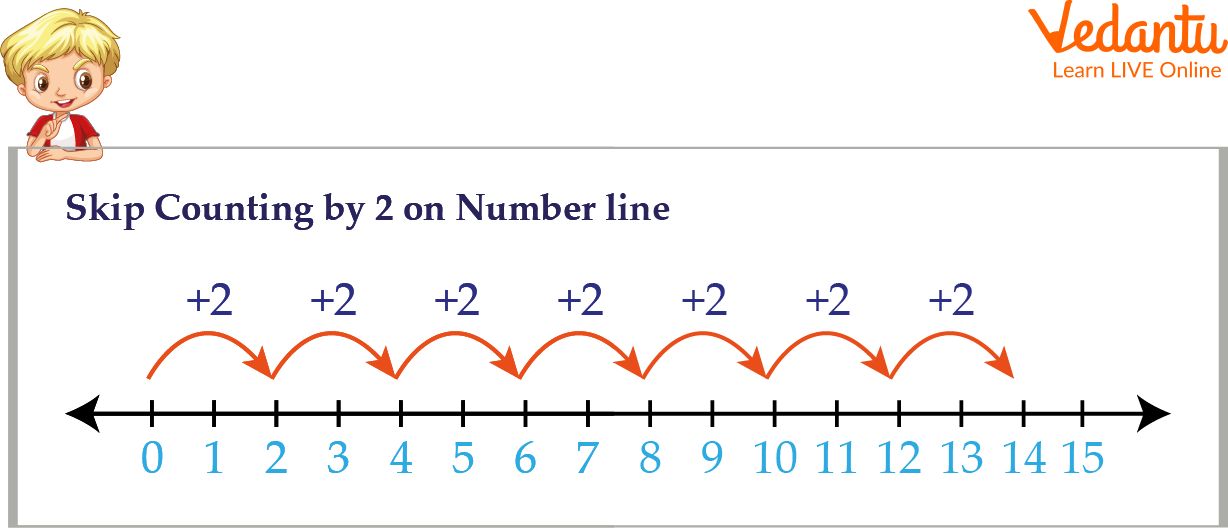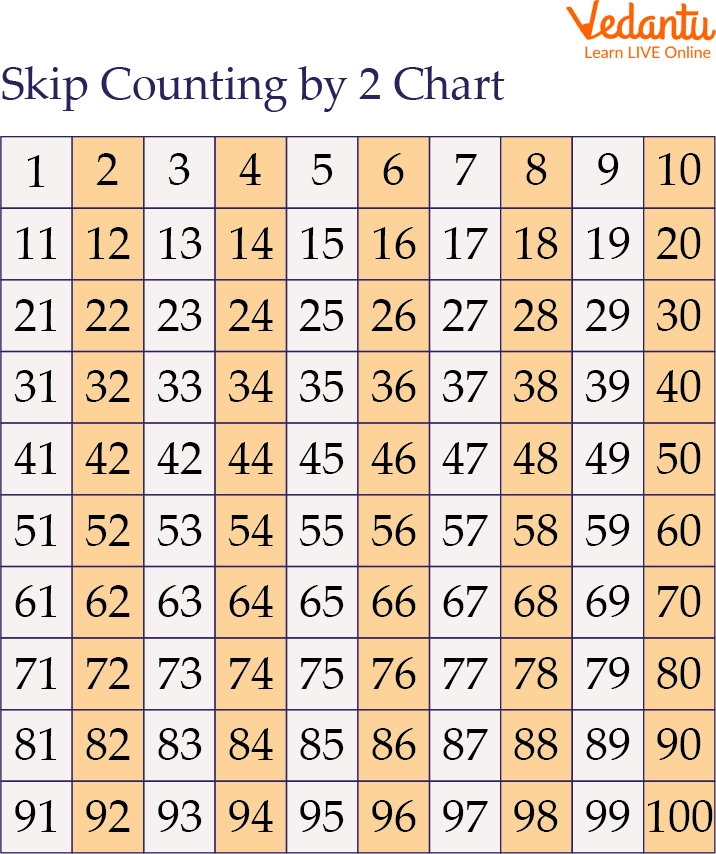Courses
Courses for Kids
Free study material
Free LIVE classes
More

# How to Skip Count in Maths## Introduction to Skip Counting

Have you ever thought about how to count by twos? This is only possible by using skip counting, an essential building block for interpreting and learning multiplication. Kids who practise skip counting will find it easier to memorise tables and deal with large numbers. This article will teach you how to count by twos, along with how we can skip counting of 2. For a deeper understanding of the idea, we will go over the steps, chart, and discussion of forward and backward skip count by 2 with the help of a few solved examples.

## What is Skip Counting?

To count forward using a number other than one is known as skip counting. Skip count is skipping a predetermined number of locations along the counting path.

Skip counting refers to adding or removing a specific amount from each successive number. To skip counting by 10, for instance, we would count as 10, 20, 30, 40, and so on. Keep in mind that each number in this series is 10 more than the one before it.

## How to Skip Count?

In this section, we will learn how to skip count by following these simple steps:

1. First, identify the beginning value.

2. Add the number skipped to the starting number to obtain the following number.

3. To find the following numbers repeat step 2.

When doing skip counting by 2 to 220. Then, we begin at 2 and skip the number by 2. So the next number becomes 4 and then moves on to the 4, continuing the same way, we get, 2, 4, 6, 8, 10……., 220. Every time, you'll see that we skipped over the numbers in between and went straight to the 2nd number after our current one.

## Types of Skip Counting

There are two types of skip counting listed below:

1. Forward Skip Counting: We count or add numbers in the forward direction to a number when we do a forward skip count. Suppose we were instructed to skip count by 2; we would do it as follows: 3, 3+2=5, 5+2=7, .… and so on.

2. Backward Skip Counting: It refers to the subtraction of a specific number. For example, starting with 100 and doing a backward skip count by 10 would involve subtracting 10 from each subsequent number, resulting in 100, 90, 80, 70, and so on.

## Skip Count by 2

Let's start by understanding how to count by twos. According to the definition of skip counting, you must keep adding the same number to the preceding one. Therefore, if we begin at 0, our next number would be 0 + 2 = 2, 2 + 2 = 4, and then 4 + 2, which equals 6, followed by 8, 10, 12, 14, 16, 18, and so on.Skip Count by 2

## Skip Counting Chart

Here is the skip counting chart:Skip Counting Chart

## Solved Examples

Q 1. Beginning at 470, write 7 numbers forward, counting by 5s.

Ans: Here, the forward skip counting has to be done. Since it’s given to skip counting by 5.

470 + 5 = 475

475 + 5 = 480

480 + 5 = 485

485 + 5 = 490

490 + 5 = 495

495 + 5 = 500

500 + 5 = 505

Therefore, the numbers are 475, 480, 485, 490, 495, 500, and 505.

Q 2. What comes next to 16 if you skip counting by 2?

Ans: By skipping two digits, we can add 2 to 16 to get the next number after 16, 16+2=18.

Therefore, if you skip count by 2, 18 comes after 16.

## Practice Problems

Q1. What comes next to 67 if you skip counting by 8?

Ans. 75.

Q2. Raml went to a magic show which had a total of 200 seats. Assume that there are 5 seats in each row and the 5th seat is a corner seat in every row. If so, what will be the seat number of the 25th row’s corner seat?

Ans: 125

Q3. Which of the following counting strategies will make the process of counting 20 bars go more quickly?

Skip counting by 1

Skip counting by 2

Ans. Skip Counting by 2.

## Summary

Let's recall what we have learned in this article. Skip counting is a method of counting that skips a predetermined number following the previous number. We can skip counting in forward and backward directions. In forward skipping, we add numbers in the forward direction to a number, while in backward skipping, we subtract numbers. You will better understand calculating 2 to 220 after going through this article. Try out the practice questions from this article on your own.

Last updated date: 28th Sep 2023
Total views: 82.5k
Views today: 1.82k

## FAQs on How to Skip Count in Maths

1. What are the advantages of using skip counting?

In mathematics, skip count helps to perform simple addition, subtraction, multiplication, and division problems. Skip counting is utilised in daily life for various tasks, including keeping score in sports, managing money when shopping, and even adding up enormous amounts. Skip count helps to save time in solving multiplication and division.

2. Describe the concept of skip counting by 3 using an example.

To skip count by three, we shall add three to the preceding figure. We shall skip two numbers in between during this process. Let's start counting from 0; the subsequent numbers will be 0 + 3 = 3, 3 + 3 = 6, 6 + 3 = 9, 9 + 3 = 12, and so on.

3. Why is it important to learn about skip counting for kids?

Skip counting has the utmost importance in daily life, with numerous concrete instances of its application. Skip counting is the practice of counting ahead or behind by a number other than one. Skip count helps to make increments of 2, 5, 10, and 100 because it is a prerequisite for number sense. Timekeeping, money handling, and scoring in basketball are a few examples of skip counting in the real world.